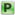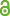Hauptmenü
• Autor
• Serdyuk, Anatoly
• Stepaniuk, Tetiana
• TitelLebesque-type inequalities for the Fourier sums on classes of generalized Poisson integrals
• Datei
• Erschienen inBulletin de la Soc. Sci. Lettres Lodz. Ser. Rech. Deform
• Erscheinungsjahr2018
• LicenceCC-BY
• Zugriffsrechte• AbstractFor functions from the set of generalized Poisson integrals $$C_β^{α,r} L_p , 1 ≤ p < ∞$$, we obtain upper estimates for the deviations of Fourier sums in the uniform metric in terms of the best approximations of the generalized derivatives $$f_β^{α,r}$$ of functions of this kind by trigonometric polynomials in the metric of the spaces $$L_p$$ . Obtained estimates are asymptotically best possible.